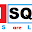# C Program To Check Number Is Divisible By 11 Or Not Using (VEDIC MATH)

Problem :- Write A C Program To Check Number Is Divisible By 11 Or Not Using (VEDIC MATH)

Logic :-
Here Is A Clarification Of  Below Program If We Want To Check Any No Is Divisible By 11 Or Not Its Very Simple You Have To Calculate Even And Odd Place Sum If Both Are Shame The No Also Will Be Divisible By 11 If Not Equal Then NOT Divisible By Now Check Code

Example :-

So take a Example 161051

So Add Even place and odd Place Numbers

Even :- 6+0+1=7

Odd :- 1+1+5=7

Now Compare Even Or Odd Number addition If Both Are Equal then Number is Divisible Else Not .

If you got a Solution then Try Below Problems .

Try Yourself C Program For Denomination of an Amount Using While Loop

Solution :

#include<stdio.h>
#include<conio.h>
void main()
{
long int r=0,i=1,odd=0,even=0,no,n,rev=0;

while(1)
{
printf("\n\nEnter Any Number : ");
scanf("%ld",&n);

no=n;

while(no!=0)
{

r=no%10;
rev=rev*10+r;
no=no/10;
}

while(rev!=0)
{
r=rev%10;
if(i%2==0)
{
even=even+r;
}
else
{
odd=odd+r;
}
rev=rev/10;
i++;
}

printf("\nOdd Digit sum = %ld \n ",odd);
printf("\nEven Digit Sum = %ld \n ",even);

if(odd==even)
printf("\n%ld Is Divisible by 11\n\n",n);
else
printf("\n%ld Is Not Divisible by 11\n\n",n);
}
getch();

}

Output:-

Previous Post
Next Post

#### post written by: Ghanendra Yadav

Hi, I’m Ghanendra Yadav, SEO Expert, Professional Blogger, Programmer, and UI Developer. Get a Solution of More Than 500+ Programming Problems, and Practice All Programs in C, C++, and Java Languages. Get a Competitive Website Solution also Ie. Hackerrank Solutions and Geeksforgeeks Solutions. If You Are Interested to Learn a C Programming Language and You Don't Have Experience in Any Programming, You Should Start with a C Programming Language, Read: List of Format Specifiers in C.

1.reverse is not require.....

2.Why you reverse the number it is totally Unnecessary !!

3.#include // line 1 //no need to reverse the no
void main()
{
int no,n=0,rem=0,n1=0;
printf("ENTER THE NO TO CHECK DIV BY 11\n"); //line 5
scanf("%d",&no);
while(no!=0)
{
rem=no%10; //taking no rem for shorting and add or sub in line 14 & 19
no=no/10; //line 10
n++;
if(n%2==0) //this function will help shorting even and odd places
{
n1=n1+rem;
printf("eve=%d %d\t",rem,n1); //line 15 //printing output to check and understand the outcome
}
else
{
n1=n1-rem; //if you do n1=rem-n1 that is {let rem=7 and 'int n1=0'} so 7-0=7 but we need 0-7=-7
printf("odd=%d %d\t",rem,n1); //line 20 //printing output to check and understand the outcome
}
}
if(n1==0)
{ printf("\n the no is div by 11\n");}
else //line 25
{ printf("\n the no is div not by 11\n");}
}